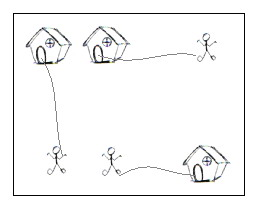# POJ2195 Going Home（二分图最优匹配）

## Going Home

• Time Limit: 1000MS
• Memory Limit: 65536K
Total Submissions: 24963
• Accepted: 12509

### Description

On a grid map there are n little men and n houses. In each unit time, every little man can move one unit step, either horizontally, or vertically, to an adjacent point. For each little man, you need to pay a \$1 travel fee for every step he moves, until he enters a house. The task is complicated with the restriction that each house can accommodate only one little man.

Your task is to compute the minimum amount of money you need to pay in order to send these n little men into those n different houses. The input is a map of the scenario, a '.' means an empty space, an 'H' represents a house on that point, and am 'm' indicates there is a little man on that point.You can think of each point on the grid map as a quite large square, so it can hold n little men at the same time; also, it is okay if a little man steps on a grid with a house without entering that house.

### Input

There are one or more test cases in the input. Each case starts with a line giving two integers N and M, where N is the number of rows of the map, and M is the number of columns. The rest of the input will be N lines describing the map. You may assume both N and M are between 2 and 100, inclusive. There will be the same number of 'H's and 'm's on the map; and there will be at most 100 houses. Input will terminate with 0 0 for N and M.

### Output

For each test case, output one line with the single integer, which is the minimum amount, in dollars, you need to pay.

### Sample Input

2 2
.m
H.
5 5
HH..m
.....
.....
.....
mm..H
7 8
...H....
...H....
...H....
mmmHmmmm
...H....
...H....
...H....
0 0

### Sample Output

2
10
28

### Source

Pacific Northwest 2004

### 链接

http://poj.org/problem?id=2195

### 题意

n个小人回到n间房子，一对一，现要使移动的总距离最少。

### 代码

StatusAccepted
Time32ms
Memory716kB
Length3171
#include <iostream>
#include <cstdio>
#include <cstring>
#include <algorithm>
using namespace std;
typedef long long ll;
const int maxn = 110;
const int inf = 0x3f3f3f3f;
int match[maxn], cx[maxn], cy[maxn], slack[maxn];
int G[maxn][maxn]; // 边权
bool visx[maxn], visy[maxn];
int nx, ny, ans;

char map[maxn][maxn];

struct Node
{
int x, y;
}nodem[maxn], nodeh[maxn];

bool find(int x)
{
int tmp;
visx[x] = 1;
for (int y = 0; y < ny; ++y)
{
if (visy[y]) // 如果这个点已经访问过
continue;
tmp = cx[x] + cy[y] - G[x][y];
if (tmp == 0) // (x, y)在相等子图中
{
visy[y] = true;
if (match[y] == -1 || find(match[y])) // 如果这个点未匹配或者能修改
{
match[y] = x;
return true;
}
}
else if (slack[y] > tmp) // (x, y)不在相等子图中
slack[y] = tmp;
}
return false;
}

void KM()
{
for (int x = 0; x < nx; ++x) // 分别对左端点进行匹配
{
for (int i = 0; i < ny; ++i) // 初始化
slack[i] = inf;
while (true)
{
memset(visx, 0, sizeof(visx)); // 初始化，每次find()更新
memset(visy, 0, sizeof(visy));
if (find(x)) // 成功匹配后换下一个
break;
int delat = inf;
for (int i = 0; i < ny; ++i) // 计算delta值
{
if (!visy[i] && delat > slack[i])
delat = slack[i];
}
for (int i = 0; i < nx; ++i)
{
if (visx[i])
cx[i] -= delat;
}
for (int j = 0; j < ny; ++j)
{
if (visy[j])
cy[j] += delat;
else
slack[j] -= delat;
}
// 修改顶标后，要把所有的slack值都减去delta
// 这是因为lx[i] 减小了delta
// slack[j] = min(lx[i] + ly[j] -w[i][j])
}
}
}

int main()
{
int r, c;
while (scanf("%d%d", &r, &c) != EOF)
{
if (r == 0 && c == 0)
return 0;
ans = 0;
int cntm = 0, cnth = 0;
for (int i = 0; i < r; ++i)
{
scanf("%s", map[i]);
for (int j = 0; j < c; ++j)
{
if (map[i][j] == 'm') // 记录小人
nodem[cntm].x = i, nodem[cntm++].y = j;
else if (map[i][j] == 'H') // 记录房子
nodeh[cnth].x = i, nodeh[cnth++].y = j;
}
}
nx = cntm, ny = cnth;
for (int i = 0; i < nx; ++i)
{
for (int j = 0; j < ny; ++j) // 边权取负数
G[i][j] = -(abs(nodem[i].x - nodeh[j].x) + abs(nodem[i].y - nodeh[j].y));
}
for (int i = 0; i < nx; ++i)
{
cx[i] = -inf;
for (int j = 0; j < ny; ++j)
{
if (cx[i] < G[i][j])
cx[i] = G[i][j];
}
}
memset(match, -1, sizeof(match));
memset(cy, 0, sizeof(cy));
KM();
for (int i = 0; i < ny; ++i)
{
if (match[i] != -1)
ans += G[match[i]][i];
}
printf("%d\n", -ans); // 答案为相反数
}
return 0;
}

The end.
2018-08-01 星期三